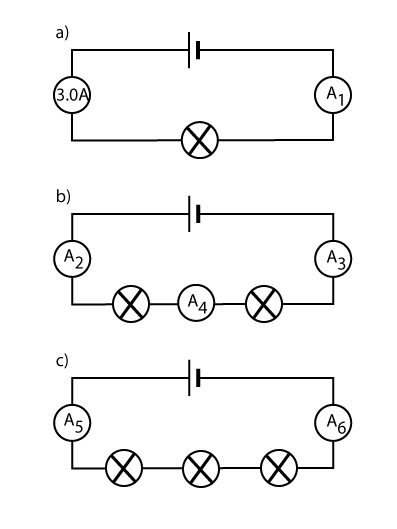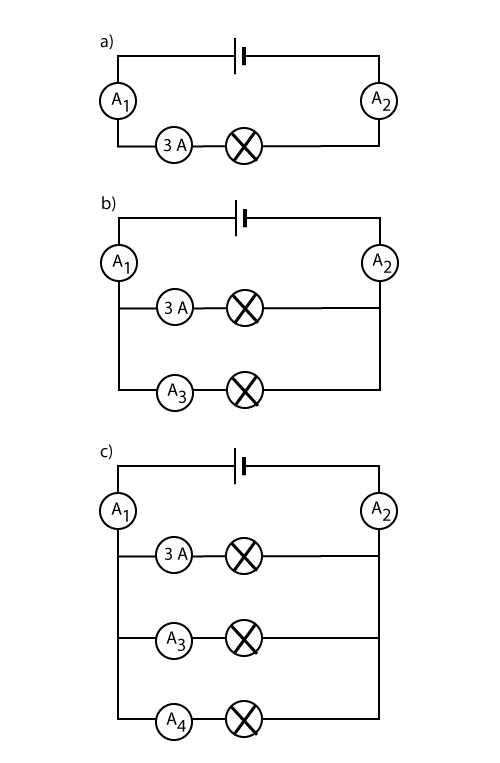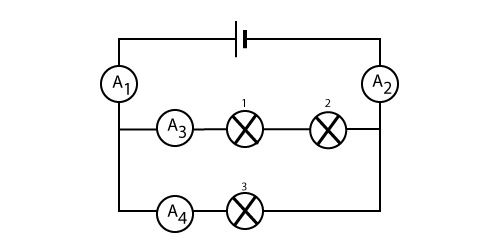# Electricity

The Learning Log

After each lesson complete a learning log at the back of your jotter. A learning log should include at least two of the following headings, especially 5): The headings should start ...
1) I really enjoyed ....
2) I want to find out more about ....
3) I was unsure about ... .
4) I was surprised by ...
5) I learned that ...

The Young Scientist's Handbook .
and see page 4.

## Homework for Lessons on Charging Up....Investigating Charge

In class today we decided to see if the size of charge on a rod depends on how many times we rub it. So each pair of us got one rod, rubbed it a different number of times and counted the pieces of paper picked up. You should have your results in your jotter, but here they are, 0, 4, 4, 8, 9, 1, 13, 8, 11 (pieces of paper).

Suggest a heading for this activity
1. What is the aim of our class experiment?
2. Write a hypothesis.
3. Draw a diagram of the apparatus we used and write one or two sentences describing what we did. Remember to mention how we controlled his variables (this means how we kept the experiment fair) and how we will measure the size of the charge.
4. i) Put our result in a table with correct headings, ii) Put our results in a line graph with correct labels.
5. Use the results to answer our aim.
6. Evaluate this experiment.
*Could we have improved it in any way?
*Is the result as you expected? If not, suggest reasons for any odd result result.
*Is there any other experiment we could do?
*Find out about at least one application of electrostatics.

## Homework for Lessons on Series and Parallel circuits.

Click on the link , solve a problem and write a report.

## Homework on drawing graphs from circuit experiments.

Click on the link and draw a couple of graphs.

Click on the link for short homework .

## The Besto Car...Watch the short clip

Help for for correctly wiring up the lights for Besto Car.

## Homework on drawing car circuit diagrams.

(a) In the clip above the car has a pair of sidelights and a pair of headlights connected to the same cell by a switch. Redraw this circuit and extend it to include a pair of brake lights. If you can, also include a pair of front windscreen wipers that come on together. Think up your own symbol for wipers and remember the brake lights and wipers need their own switch. The usual rule applies that if one brake light fails or if one wiper malfunctions the other is in no way affected. You can include any other device you wish. If you need a larger piece of paper for this task then this can be provided. Always use a ruler and maybe your work can be displayed on the wall.

(b) If you know how, and only if, show how the rectangular frame of the car can be used in the circuit. This is partly done in the clip.

## Investigating Resistance.

Investigative scientists are always clear on what it is they are changing and what they are measuring or observing. We give these quantities different terms. What we change is called the independent variable and what we observe is called the dependent variable.
i) What is the independent variable in the circuit below?
ii) What are the dependant variables in the circuit below?

## Revising Circuits.

Series Circuit Facts
We learned there are two types of circuit: Series and Parallel. What we learned:
i) In a series circuit the current is the same at all points.
ii) The more bulbs in series then the dimmer they will be.
iii)The more bulbs in series then the lower the current will be.

1) Find the current in each ammeter below (if we use twice as many bulbs then the current will half and so on).
2) What bulb will be the brightest?Parallel Circuit Facts
We learned there are two types of circuit: Series and Parallel. What we learned:
i) In a parallel circuit the current in each bulb adds up to the total current.
ii) More or less bulbs in parallel does not affect their brightness.
iii) If we add more bulbs in parallel then the total current increases.

1) Find the current in each ammeter below (if we use twice as many bulbs then the current will double and so on).
2) What bulb will be the brightest? This is a trick question.Harder Question
Find the current in the ammeters below and find the brightest bulbBring your answers to me tomorrow and I'll mark them before the test!